Next: Infinite body, rectangular coordinate Up: Heat Equation. Transient Heat Previous: Heat Equation. Transient Heat

## Rectangular coordinates. Transient 1-D.

The Heat Equation for 1-D transient Green's Functions in rectangular coordinates is: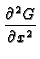+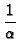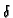(x - x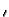)(t -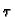) =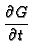Kevin D. Cole
2002-12-31